Next: Frequentistic coverage Up: Appendix on probability and Previous: Are the beliefs in   Contents

# Biased Bayesian estimators and Monte Carlo checks of Bayesian procedures

This problem has already been raised in Sectionsand. We have seen there that the expected value of a parameter can be considered, somehow, to be analogous to the estimators8.10 of the frequentistic approach. It is well known, from courses on conventional statistics, that one of the nice properties an estimator should have is that of being free of bias.

Let us consider the case of Poisson and binomial distributed observations, exactly as they have been treated in Sectionsand, i.e. assuming a uniform prior. Using the typical notation of frequentistic analysis, let us indicate with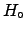the parameter to be inferred, withits estimator.

Poisson: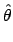;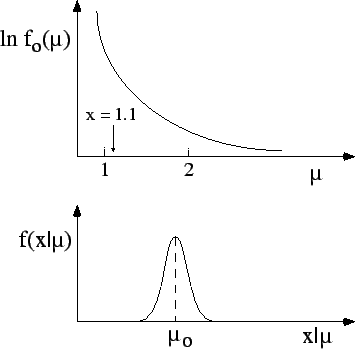indicates the possible observation andis the estimator in the light of: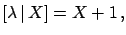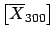E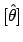EE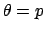(8.3)

The estimator is biased, but consistent (the bias become negligible whenis large).
Binomial:; after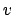trials one may observefavourable results, and the estimator of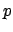is thenE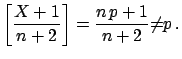EE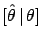(8.4)

In this case as well the estimator is biased, but consistent.
What does it mean? The result looks worrying at first sight, but, in reality, it is the analysis of bias that is misleading. In fact:
• the initial intent is to reconstruct at best the parameter, i.e. the true value of the physical quantity identified with it;
• the freedom from bias requires only that the expected value of the estimator should equal the value of the parameter, for a given value of the parameter,
 E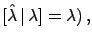e.g.    E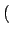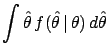i.e.(8.5)

But what is the true value of? We don't know, otherwise we would not be wasting our time trying to estimate it (always keep real situations in mind!). For this reason, our considerations cannot depend only on the fluctuations ofaround, but also on the different degrees of belief of the possible values of. Therefore they must depend also on. For this reason, the Bayesian result is that which makes the best use8.11 of the state of knowledge aboutand of the distribution offor each possible value. This can be easily understood by going back to the examples of Section. It is also easy to see that the freedom from bias of the frequentistic approach requiresto be uniformly distributed from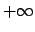to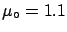(implicitly, as frequentists refuse the very concept of probability of). Essentially, whenever a parameter has a limited range, the frequentistic analysis decrees that Bayesian estimators are biased.

There is another important and subtle point related to this problem, namely that of the Monte Carlo check of Bayesian methods. Let us consider the case depicted in Fig.and imagine making a simulation, choosing the value, generating many (e.g. 10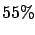000) events, and considering three different analyses:

1. a maximum likelihood analysis;
2. a Bayesian analysis, using a flat distribution for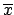;
3. a Bayesian analysis, using a distribution of`of the kind'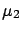of Fig., assuming that we have a good idea of the kind of physics we are doing.
Which analysis will reconstruct a value closest to? You don't really need to run the Monte Carlo to realize that the first two procedures will perform equally well, while the third one, advertised as the best in these notes, will systematically underestimate!

Now, let us assume we have observed a value of, for example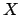. Which analysis would you use to infer the value of? Considering only the results of the Monte Carlo simulation it seems obvious that one should choose one of the first two, but certainly not the third!

This way of thinking is wrong, but unfortunately it is often used by practitioners who have no time to understand what is behind Bayesian reasoning, who perform some Monte Carlo tests, and decide that the Bayesian theorem does not work!8.12 The solution to this apparent paradox is simple. If you believe thatis distributed likeof Fig., then you should use this distribution in the analysis and also in the generator. Making a simulation based only on a single true value, or on a set of points with equal weight, is equivalent to assuming a flat distribution forand, therefore, it is not surprising that the most grounded Bayesian analysis is that which performs worst in the simple-minded frequentistic checks. It is also worth remembering that priors are not just mathematical objects to be plugged into Bayes' theorem, but must reflect prior knowledge. Any inconsistent use of them leads to paradoxical results.Next: Frequentistic coverage Up: Appendix on probability and Previous: Are the beliefs in   Contents
Giulio D'Agostini 2003-05-15